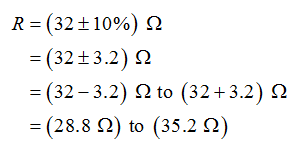# You have 100-ohm resistors. Design a 32-ohm resistor using them. The resistor should be 10% resistance. How would you do that?

Question
10 views
You have 100-ohm resistors. Design a 32-ohm resistor using them. The resistor should be 10% resistance. How would you do that?
check_circle

Step 1

The range of the resistance for the resistor that to be designed can be considered as,Step 2

From the above, it is clear that the resistance for the resistor that to be designed should lie between the range 28.8 Ω to 35.2 Ω. Thus, consider the three resistors of 100 Ω and connect these in parallel combination.

Use the expression for the equivalent resistance of the resistors in parallel combination, solve...

### Want to see the full answer?

See Solution

#### Want to see this answer and more?

Solutions are written by subject experts who are available 24/7. Questions are typically answered within 1 hour.*

See Solution
*Response times may vary by subject and question.
Tagged in